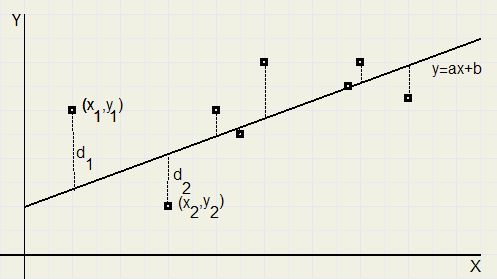Linear RegressionIntroduction
In this article I present a nice formula for the regression line though a set of points in a plane.

Given are points (xi, yi)...where i = 1,2,...,n
Asked the line y = ax + b which has the least deviation with these points.A commom measure for the deviation is the sum of squares of the differences:
d12 + d22 + ..... + dn2
in case of n points.

For point i we have :
di = yi - (axi + b)
Before continuing, first some definitions and rules:

Definition
sum:
S xi = x1+ x2 + .... + xn

average:
 x
=
 Σ xi
n
Arithmetic rules
S (xi + yi) = S xi + S yi

if c is a constant:

S cxi = c S xi
and
S c = n c

from the average we conclude:
 Σ xi
= n  x
application of the rules:
Σ  x
 y
= n  x
 y
=  y
 Σ xi
The formula's for a and b of the regression line y = ax + b
Function f(a,b) of the sum of the squared deviations of points 1..n is:
 f (a , b)
=  Σ (yi − (a xi + b)) 2
f(a,b) is first differentiated to a , then to b.

differentiation to a:
f 'a(a,b) = 2  Σ (yi − (a xi + b)) · −xi
differentiation to b:
f 'b(a,b) = 2  Σ (yi − (a xi + b)) · −1
For the best fit, both derivatives must be zero.
This yields the following system of equations::
 Σ (xi yi − a xi 2 − b xi)
= 0
...................1)
 Σ (yi − a xi − b)
= 0
....................2)
from ....2) we see
 Σ yi
− a  Σ xi
− b n = 0

 Σ yi
n
−
a  Σ xi
n
− b = 0

b =  y
− a  x
................3)
substitute result for b at ........1)
Σ (xi yi − a xi 2 − ( y
− a  x
) xi)
= 0

Σ (xi yi − a xi 2 −  y
xi + a  x
xi)
= 0

 Σ xi yi
− a  Σ xi 2
−  y
 Σ xi
+ a  x
 Σ xi
= 0

 Σ xi yi
− a ( Σ xi 2
−  x
 Σ xi
) −  y
 Σ xi
= 0

a ( Σ xi 2
−  x
 Σ xi
) =  Σ xi yi
−  y
 Σ xi

a =
 Σ xi yi
−  y
 Σ xi
 Σ xi 2
−  x
 Σ xi
Formally, we have found the formula's for a and b.
Above value of a can be substituted at .......3) to know b.
However, with some manipulation the formula may be converted into a more elegant form.
We separately attack nominator and denominator.

1. the nominator
 Σ xi yi
−  y
 Σ xi
=
Σ (xi yi −  y
xi −  x
 y
+  x
 y
)
=
Σ (xi yi −  y
xi −  x
yi +  x
 y
)
=
Σ (xi −  x
)
· (yi −  y
)
2. the denominator
 Σ xi 2
−  x
 Σ xi
=
Σ (xi 2 −  x
xi)
=
Σ (xi 2 − 2  x
xi +  x
xi)
=
Σ (xi 2 − 2  x
xi + ( x
) 2)
=
Σ (xi −  x
) 2
summarizing:
a =
Σ (xi −  x
)
· (yi −  y
)
Σ (xi −  x
) 2

b =  y
− a  x

Note:
please look [here] for an article about the best polynomial through a set of points.
It is a nice application of linar algebra.